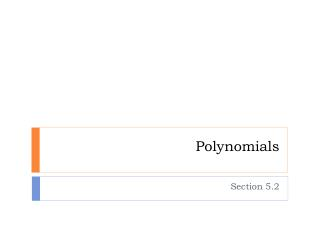DownloadDownload PresentationPolynomials

# Polynomials

Télécharger la présentation## Polynomials

- - - - - - - - - - - - - - - - - - - - - - - - - - - E N D - - - - - - - - - - - - - - - - - - - - - - - - - - -
##### Presentation Transcript

1. Polynomials Section 5.2

2. Looking back…

3. Definitions • Polynomial: Monomial or sum of monomials • Example: 7a2 + b + 6ac2 - 8ac • Like Terms: 2 monomials that are the same or differ only by their coefficients. • Example: 2x and 4x 3y and 3y • Terms: Monomials that make up polynomials

4. Identifying Polynomial • Monomials contain variables whose exponents can be written as whole numbers. • Is it a polynomial? • 7a2+ b + 6ac2 - 8ac • Yes, each monomial (term) has variables whose exponents are whole numbers

5. Is it a polynomial?

6. Degree • The degree of a polynomial is the highest degree among all terms.

7. Simplify and identify the degree

8. Simplify and identify the degree

9. Simplify and identify the degree

10. Simplify and identify the degree